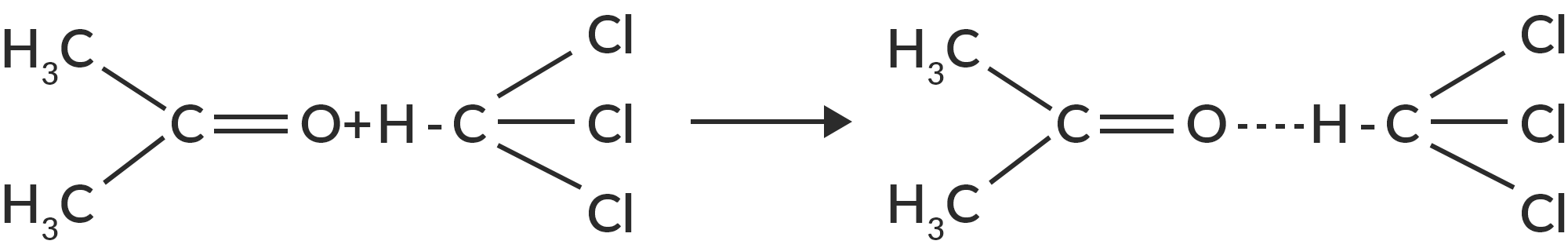Enthalpy Change for the Interaction between Acetone and Chloroform

# Our Objective

Enthalpy change for the interaction between acetone and chloroform.

# The Theory

Thermodynamics is the study of the connection between heat and work, which explains heat characteristics and heat energy reactions with various molecules. Enthalpy is the critical and fundamental element that determines whether or not a reaction may occur. Enthalpy change is the amount of heat that enters or exits a system during a reaction. Because of the creation of hydrogen bonds between chloroform and acetone, acetone produces heat when combined with chloroform. The heat generated during this contact may be quantified by mixing these two liquids and measuring the enthalpy change with a calorimeter.

## Enthalpy Change Definition

The enthalpy change definition can be explained in several ways. We can explain the sum of both internal energy and the product of volume and pressure in simple terms.

H= E+ PV

Where H = Enthalpy change

E = Internal Energy

P = Pressure

V = Volume

Raoult's law was named after François-Marie Raoult, a French chemist who discovered, while conducting an experiment, that when chemicals were combined in a solution, the solution's vapour pressure reduced concurrently. Raoult's law, which was developed in 1887, is also known as the law of thermodynamics.

Raoult's law says that the partial vapour pressure of a solvent in a solution (or mixture) is equal to or identical to the pure solvent's vapour pressure multiplied by its mole fraction in the solution.

Raoult's law equation is written mathematically as;

Psolution = ΧsolventP0solvent

Where,

Psolution = vapour pressure of the solution
Χsolvent  = mole fraction of the solvent
P0solvent = vapour pressure of the pure solvent

When liquid pairs combine, they deviate from ideal behaviour. Acetone and chloroform combine to generate a non-ideal liquid pairing system that deviates from the Raoult law. Acetone-chloroform combination is a well-known example of a system that deviates significantly from the behaviour of the ideal solution. As a result, it was the focus of several theoretical and experimental studies.This hydrogen bonding reduces the escape tendency of both molecules, resulting in a lower vapour pressure than indicated by Raoult's equation. In the pure state, however, only the weak Van der Waal forces maintain both chloroform and acetone molecules.

The creation of hydrogen bonds causes a change in enthalpy in this process. The heat change for the provided amount is recorded. As a result, when 1 mol chloroform is combined with 1 mol acetone, enthalpy changes are observed.

ΔH1 Heat gained by calorimeter, thermometer and stirrer + ΔH2 Enthalpy change for chloroform + ΔH3 Enthalpy change for acetone + ΔH4 Enthalpy change for interaction =0

∴ΔH4 = -ΔH1 + ΔH2 + ΔH3

# Learning Outcomes

• Students learn the terms ideal solution and deviation from ideal solution.
• Students learn the term enthalpy.
• They learn the laboratory skills.